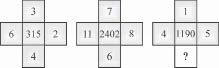# User ForumSubject :IMO    Class : Class 9

A set of figures carrying certain characters, is given. Assuming that the characters in each set follow a similar pattern, then find the missing character.A1
B2
C6
D10

what is the solution of this question??

Class : Class 10

## Ans 2:

Class : Class 9
Solve- In 1st figure, 6^3+4^3+3^3+2^3=315 ​ In 2nd figure, 11^3+8^3+7^3+6^3=2402 Similarly, in 3rd figure, 5^3+4^3+1^3+x^3=1190 = 190+x^3=1190 = x^3=1000 = x=10 So, correct answer is D) 10

Class : Class 10
how

Class : Class 9